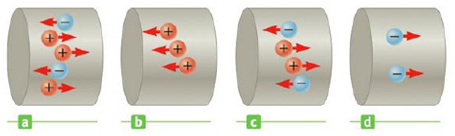Chapter 17.1, Problem 17.1QQ

Chapter
Section
Textbook Problem

Consider positive and negative charges all moving horizontally with the same speed through the four regions in Figure 17.2. Rank the magnitudes of the currents in these four regions from lowest to highest. (Ia is the current in Figure 17.2a, Ib the current in Figure 17.2b, etc.) (a) Id, Ia, Ic, Ib (b) Ia, Ic, Ib, Id (c) Ic, Ia, Id, Ib (d) Id, Ib, Ic, Ia (e) Ia, Ib, Ic, Id (f) None of theseFigure 17.2(Quick Quiz 17.1)

To determine
The order of magnitudes of the currents from lowest to highest.

Explanation

Given Info: Positive and negative charges all moving horizontally with the same speed through four regions in figure 17.2

Current is the rate of flow of electric charge. Negative charge moving towards left is same as positive charge moving towards right.

Use the principle of addition of charges to calculate the amount of charge flowing.

1. (a) Positive 3 charges moving towards right and negative 2 charges moving towards left 3+2=5 net charge.
2. (b) Positive 3 charges moving towards right =3 net charge.
3. (c) Positive 3 charges moving towards right and negative 2 charges moving towards left 2+2=4 net charge.
4. (d) Negative 2 charges moving towards right =2 net charge

Still sussing out bartleby?

Check out a sample textbook solution.

See a sample solution

The Solution to Your Study Problems

Bartleby provides explanations to thousands of textbook problems written by our experts, many with advanced degrees!

Get Started

Find more solutions based on key concepts